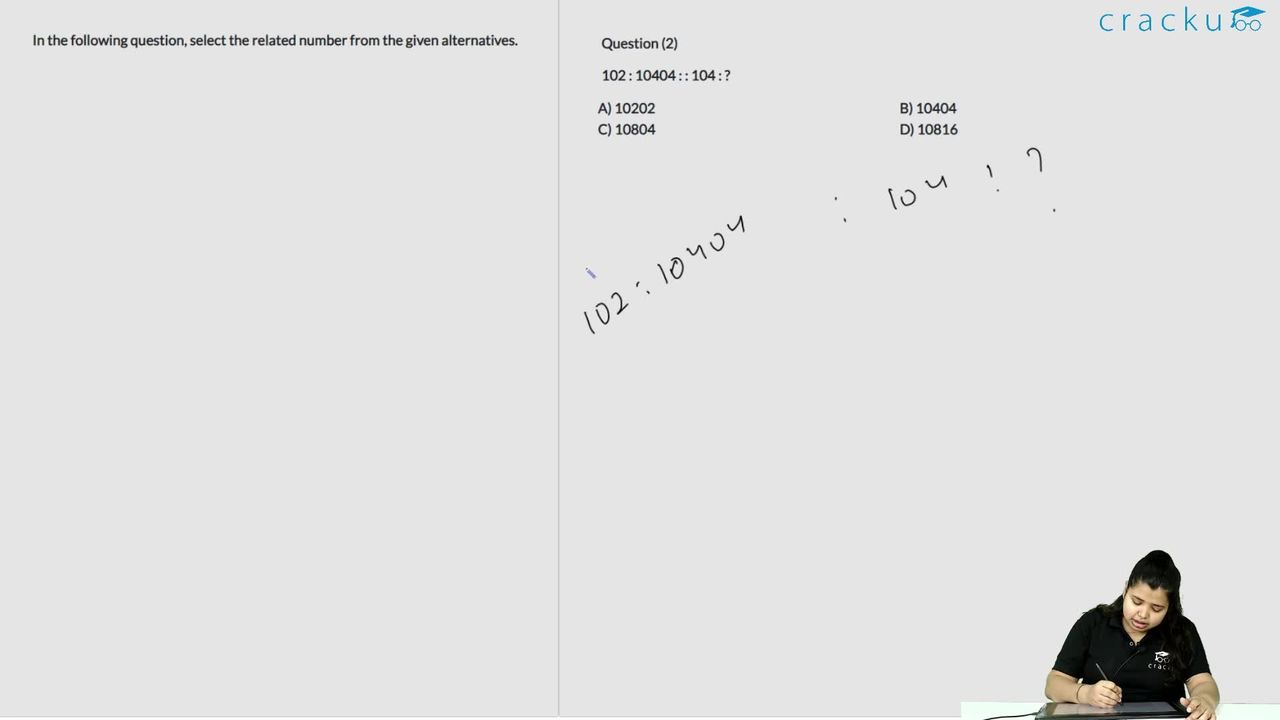### SSC CPO 3 July 2017 Afternoon Shift Question 6

Instructions

In the following question, select the related number from the given alternatives.

Question 6

# 102 : 10404 : : 104 : ?

Solution

Expression = 102 : 10404 : : 104 : ?

The pattern followed is = $$n:(n)^2$$

Eg :- $$(102)^2=10404$$

Similarly, $$(104)^2=10816$$

=> Ans - (D)

### View Video Solution